38 Assigning Names to Things
Do a simple computation:
 In:=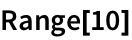Out=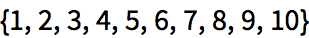% gives the result of the previous computation:
 In:=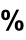Out=This squares the most recent result:
 In:=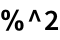Out=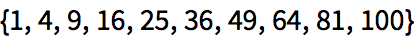Assign thing to be the result of Range:
 In:=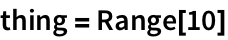Out=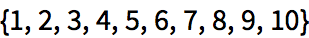Whenever thing appears, itll be replaced by the result of Range:
 In:=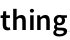Out=Square the value of thing:
 In:=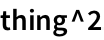Out=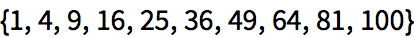Assign a name to a list of a million elements, but dont display the list:
 In:=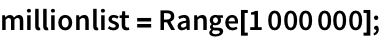In:=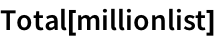Out=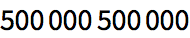When you assign a name to something, the name will stay until you explicitly clear it.
Assign x the value 42:
 In:=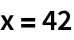Out=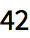You might have thought this would be {x, y, z}but x has value 42:
 In:=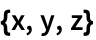Out=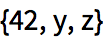Clear any assignment for x:
 In:=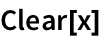Now x, like y and z, isnt replaced:
 In:=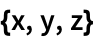Out=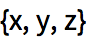In:=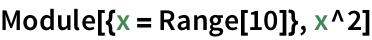Out=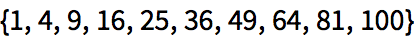Outside the module, x still has no value assigned:
 In:=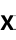Out=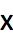Define local variables x and n, then compute x^n using the values youve assigned:
 In:=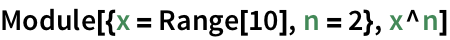Out=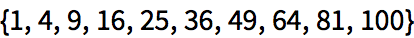To specify sequences of actions in the Wolfram Language one just separates them by semicolons (;). (Putting a semicolon at the end is like specifying an empty final action, which is why this has the effect of not displaying a result.)
Do a sequence of operations; the result is what the last operation produces:
 In:=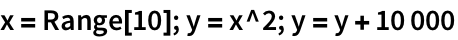Out=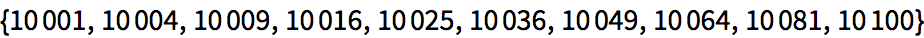This defined global values for x and y; dont forget to clear them:
 In:=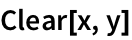You can use semicolons to do sequences of operations inside Module.
This does a sequence of operations, with x and y maintained as local variables:
 In:=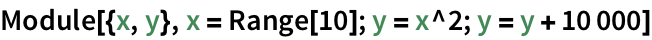Out=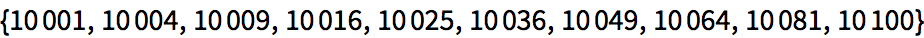You can mix local variables that do and dont have initial values:
 In:=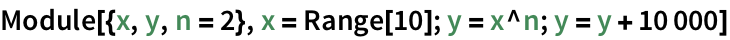Out=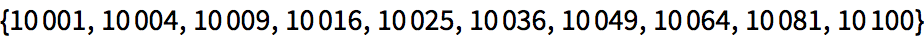% the most recent computation result x=value assign a value Clear[x] clear a value Module[{x=value}, ...] set up a temporary variable expr; do a computation, but don’t display its result expr1; expr2; ... do a sequence of computations
38.1Use Module to compute x^2+x where x is Range»
Expected output:
 Out[]=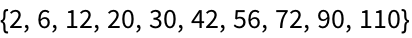Answer & check your solution
38.2Use Module to generate a list of 10 random integers up to 100, then make a column giving the original list, and the results of applying Sort, Max and Total to it. »
Sample expected output:
 Out[]=Answer & check your solution
38.3Use Module to generate an image collage from a picture of a giraffe, and the results of applying Blur, EdgeDetect and ColorNegate to it. »
Sample expected output:
 Out[]=Answer & check your solution
38.4Inside a Module, let r=Range, then make a line plot of r joined with the reverse of r joined with r joined with the reverse of r»
Expected output:
 Out[]=Answer & check your solution
38.5Find a simpler form for {Range+1, Range-1, Reverse[Range]}»
Expected output:
 Out[]=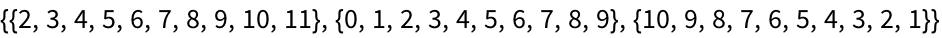Answer & check your solution
38.6Find a simpler form for Module[{u=10}, Join[{u}, Table[u=Mod[17u+2, 11], 20]]]»
Expected output:
 Out[]=Answer & check your solution
38.7Generate 10 random strings made of 5 letters, in which consonants (non-vowels) alternate with vowels (aeiou). »
Sample expected output:
 Out[]=Answer & check your solution
How come were at Section 38, and only now introducing variable assignments?
Because, as weve seen, in the Wolfram Language one can go a very long way without introducing them. And it tends to be a lot cleaner to program without them.
Can I assign a name to anything?
Yes. Graphics, arrays, images, pure functions, whatever.
x equals 4, or, more rarely, assign x to 4, or give x the value 4.
Use names that are specific and explicit. Dont worry if theyre long; theyll get autocompleted when you type. For informal names, start with lowercase letters. For more carefully thought out names, consider using capitalization like built-in functions do.
% gives the previous result. What about the result before that, etc.?
%% gives the next to last, %%% gives the next-to-next-to-last, etc. %n gives the result on line n (i.e. the result labeled Out[n]).
Yes. x=y=6 assigns both x and y to 6. {x, y}={3, 4} assigns x to 3 and y to 4. {x, y}={y, x} swaps the values of x and y.
What happens if a variable escapes from a Module without having been assigned a value?
Try it! Youll find youll get a new variable thats been given a unique name.
What about other procedural programming constructs, like Do and For?
The Wolfram Language has those. Do is sometimes worth using, particularly when your objective is side effects, like assigning variables or exporting data. For is almost always a bad idea, and can almost always be replaced by much cleaner code using constructs such as Table.